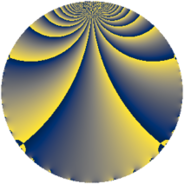# Properties

 Label 182.2.iLevel $182$ Weight $2$ Character orbit 182.i Rep. character $\chi_{182}(83,\cdot)$ Character field $\Q(\zeta_{4})$ Dimension $24$ Newform subspaces $1$ Sturm bound $56$ Trace bound $0$

# Learn more

## Defining parameters

 Level: $$N$$ $$=$$ $$182 = 2 \cdot 7 \cdot 13$$ Weight: $$k$$ $$=$$ $$2$$ Character orbit: $$[\chi]$$ $$=$$ 182.i (of order $$4$$ and degree $$2$$) Character conductor: $$\operatorname{cond}(\chi)$$ $$=$$ $$91$$ Character field: $$\Q(i)$$ Newform subspaces: $$1$$ Sturm bound: $$56$$ Trace bound: $$0$$

## Dimensions

The following table gives the dimensions of various subspaces of $$M_{2}(182, [\chi])$$.

Total New Old
Modular forms 64 24 40
Cusp forms 48 24 24
Eisenstein series 16 0 16

## Trace form

 $$24q + 8q^{7} - 40q^{9} + O(q^{10})$$ $$24q + 8q^{7} - 40q^{9} + 4q^{14} + 16q^{15} - 24q^{16} + 8q^{18} + 4q^{21} + 8q^{22} + 8q^{28} - 16q^{29} + 20q^{35} - 8q^{37} - 40q^{39} + 12q^{42} - 24q^{50} + 16q^{53} + 16q^{57} + 8q^{58} - 16q^{60} - 40q^{63} + 88q^{65} - 96q^{67} - 44q^{70} - 64q^{71} - 8q^{72} - 16q^{74} + 16q^{78} - 8q^{79} + 168q^{81} + 4q^{84} - 56q^{85} + 56q^{86} + 44q^{91} - 8q^{92} + 112q^{93} + 32q^{98} - 16q^{99} + O(q^{100})$$

## Decomposition of $$S_{2}^{\mathrm{new}}(182, [\chi])$$ into newform subspaces

Label Dim. $$A$$ Field CM Traces $q$-expansion
$$a_2$$ $$a_3$$ $$a_5$$ $$a_7$$
182.2.i.a $$24$$ $$1.453$$ None $$0$$ $$0$$ $$0$$ $$8$$

## Decomposition of $$S_{2}^{\mathrm{old}}(182, [\chi])$$ into lower level spaces

$$S_{2}^{\mathrm{old}}(182, [\chi]) \cong$$ $$S_{2}^{\mathrm{new}}(91, [\chi])$$$$^{\oplus 2}$$